# Calculating weekdays in Excel - WORKDAY and NETWORKDAYS functions

This short tutorial explains the use of Excel NETWORKDAYS and WORKDAY functions to calculate workdays with custom weekend parameters and holidays.

Microsoft Excel provides two functions specially designed for calculating weekdays - WORKDAY and NETWORKDAYS.

The WORKDAY function returns a date N working days in the future or in the past and you can use it to add or subtract workdays to a given date.

Using the NETWORKDAYS function, you can calculate the number of workdays between two dates that you specify.

In modem versions of Excel 2010, 2013 as well as coming Excel 2016, more powerful modifications of the above-said functions are available, WORKDAY.INTL and NETWORKDAYS.INTL, which let you define which and how many days are weekend days.

And now, let's have a closer look at each function and see how you can use it to calculate working days in your Excel worksheets.

## Excel WORKDAY function

The Excel WORKDAY function returns a date that is a given number of working days ahead of or prior to the start date. It excludes weekends as well as any holidays you specify.

The WORKDAY function is purposed for calculating workdays, milestones and due dates based on the standard working calendar, with Saturday and Sunday being the weekend days.

WORKDAY is a built-in function in Excel 2007, 2010, 2013 and Excel 2016. In earlier versions, you need to enable the Analysis ToolPak.

When using WORKDAY in Excel, you have to input the following arguments:

WORKDAY(start_date, days, [holidays])

The first 2 arguments are required and the last one is optional:

• Start_date - the date from which to start counting weekdays.
• Days - the number of workdays to add to / subtract from start_date. A positive number returns a future date, a negative number returns a past date.
• Holidays - an optional list of dates that should not to be counted as working days. This can be either a range of cells containing the dates you want to exclude from calculations, or an array constant of the serial numbers representing the dates.

Now that you know the basics, let's see how you can use the WORKDAY function in your Excel worksheets.

#### How to use WORKDAY to add / subtract business days to date

To calculate workdays in Excel, follow these simple rules:

• To add workdays, enter a positive number as the days argument of a WORKDAY formula.
• To subtract workdays, use a negative number in the days argument.

Supposing you have a start date in cell A2, a list of holidays in cells B2:B5, and you want to find out the dates 30 workdays in the future and past. You can do this using the following formulas:

To add 30 workdays to the start date, excluding holidays in B2:B5:

`=WORKDAY(A2, 30, B2:B5)`

To subtract 30 workdays from the start date, excluding holidays in B2:B5:

`=WORKDAY(A2, -30, B2:B5)`

To calculate weekdays based on the current date, use the TODAY() function as the start date:

To add 30 workdays to today's date:

`=WORKDAY(TODAY(), 30)`

To subtract 30 workdays from today's date:

`=WORKDAY(TODAY(), -30)`

To supply the start date directly to the the formula, use the DATE function:

`=WORKDAY(DATE(2015,5,6), 30)`

The following screenshot demonstrates the results of all these and a few more WORKDAY formulas: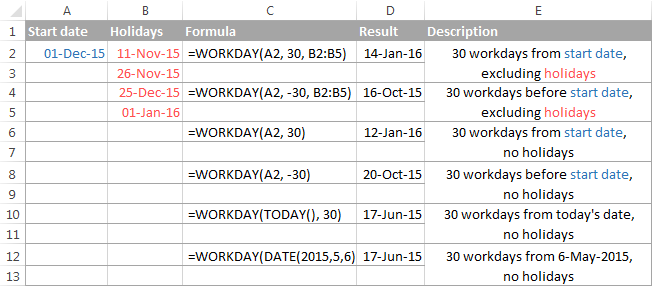And naturally, you can enter the number of workdays to add to / subtract from the start date in some cell, and then refer to that cell in your formula. For example:

`=WORKDAY(A2, C2)`

Where A2 is the start date and C2 is the number of non-weekend days behind (negative numbers) or ahead of (positive numbers) the start date, no holidays to exclude.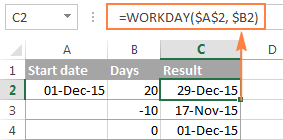## Excel WORKDAY.INTL function

WORKDAY.INTL is a more powerful modification of Excel WORKDAY function that works with custom weekend parameters. As well as WORKDAY, it returns a date that is a specified number of workdays in the future or in the past, but lets you determine which days of the week should be considered weekend days.

The WORKDAY.INTL function was introduced in Excel 2010 and so is not available in earlier Excel versions.

The syntax of the Excel WORKDAY.INTL function is as follows:

WORKDAY.INTL(start_date, days, [weekend], [holidays])

The first two arguments are required and are akin to WORKDAY's:

Start_date - the initial date.

Days - the number of working days before (negative value) or after (positive value) the start date. If the `days` argument is supplied as a decimal number, it is truncated to the integer.

The last two arguments are optional:

Weekend - specifies which weekdays should be counted as weekend days. This can be either a number or a string, as demonstrated below.

 Number Weekend days 1 or omitted Saturday, Sunday 2 Sunday, Monday 3 Monday, Tuesday 4 Tuesday, Wednesday 5 Wednesday, Thursday 6 Thursday, Friday 7 Friday, Saturday 11 Sunday only 12 Monday only 13 Tuesday only 14 Wednesday only 15 Thursday only 16 Friday only 17 Saturday only

Weekend string - a series of seven 0's and 1's that represent seven days of the week, beginning with Monday. 1 represents a non-working day and 0 represents a workday. For example:

• "0000011" - Saturday and Sunday are weekends.
• "1000001" - Monday and Sunday are weekends.

At first sight, weekend strings may seem superfluous, but I personally like this method better because you can make a weekend string on the fly without having to remember any numbers.

Holidays - an optional list of dates you want to exclude from the working day calendar. This can be a range of cells containing the dates, or an array constant of the serial values representing those dates.

#### Using WORKDAY.INTL in Excel - formula examples

Well, the pretty big bulk of theory we've just discussed may seem quite complicated and confusing, but trying your hand at formulas will make things really easy.

On our dataset, with the start date in cell A2 and a list of holidays in A5:A8, let's calculate workdays with custom weekends.

• To add 30 workdays to the start date, Friday and Saturday counted as weekends and holidays in A5:A8 excluded:`=WORKDAY.INTL(A2, 30, 7, A5:A8)`
or
`=WORKDAY.INTL(A2, 30, "0000110", A5:A8)`
• To subtract 30 workdays from the start date, Sunday and Monday counted as weekends and holidays in A5:A8 excluded:`=WORKDAY.INTL(A2, -30, 2, A5:A8)`
or
`=WORKDAY.INTL(A2, -30, "1000001", A5:A8)`
• To add 10 workdays to the current date, Sunday being the only weekend day, no holidays:`=WORKDAY.INTL(TODAY(), 10, 11)`
or
`=WORKDAY.INTL(A2, 10, "0000001")`

In your Excel sheet, the formulas may look similar to this:Note. Both Excel WORKDAY and WORKDAY.INTL functions return serial numbers representing the dates. To get those numbers displayed as dates, select the cells with the numbers and press Ctrl+1 to open the Format Cells dialog. On the Number tab, select Date in the Category list, and choose the date format you want. For the detailed steps, please see How to change date format in Excel.

### Excel WORKDAY and WORKDAY.INTL errors

If your Excel WORKDAY or WORKDAY.INTL formula returns an error, the reason is likely to be one of the following:

#NUM! error occurs if either:

• a combination of the `start_date` and `days` arguments results in an invalid date, or
• `weekend` argument in the WORKDAY.INTL function is invalid.

#VALUE! error occurs if either:

• `start_date` or any value in `holidays` is not a valid date, or
• `days` argument is non-numeric.

## Excel NETWORKDAYS function

The NETWORKDAYS function in Excel returns the number of workdays between two dates, excluding weekends and, optionally, the holidays you specify.

The syntax of Excel NETWORKDAYS is intuitive and easy-to-remember:

NETWORKDAYS(start_date, end_date, [holidays])

The first two arguments are obligatory and the third one is optional:

• Start_date - initial date from which to start counting working days.
• End_date - the end of the period for which you are counting workdays.

Both the start date and end date are counted in the returned number of workdays.

• Holidays - an optional list of holidays that should not to be counted as work days.

#### How to use NETWORKDAYS in Excel - formula examples

Let's say you have a list of holidays in cells A2:A5, start dates in column B, end dates in column C, and you want to know how many workdays are between these dates. The appropriate NETWORKDAYS formula is easy to figure out:

`=NETWORKDAYS(B2, C2, \$A\$2:\$A\$5)`

Notice that the Excel NETWORKDAYS function returns a positive value when the start date is less than the end date, and a negative value if the end date is more recent than the start date (as in row 5):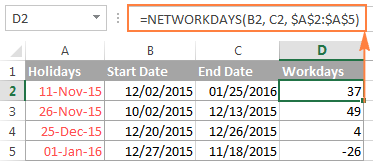## Excel NETWORKDAYS.INTL function

Like NETWORKDAYS, Excel's NETWORKDAYS.INTL function calculates the number of weekdays between two dates, but lets you specify which days should be counted as weekend days.

The syntax of the NETWORKDAYS.INTL function is very similar to NETWORKDAYS', except it has the additional [weekend] parameter that indicates which days of the week should be counted as weekends.

NETWORKDAYS.INTL( start_date, end_date, [weekend], [holidays] )

The `weekend` argument can accept either a number or a string. The numbers and weekend strings are exactly the same as in the `weekend` parameter of the WORKDAY.INTL function.

The NETWORKDAYS.INTL function is available in modem versions of Excel 2010, 2013 and Excel 2016.

#### Using NETWORKDAYS.INTL in Excel - formula examples

Using the list of dates from the previous example, let's calculate the number of workdays between two dates with Sunday being the only weekend day. For this, you type number 11 in the `weekend` argument of your NETWORKDAYS.INTL formula or make a string of six 0's and one 1 ("0000001"):

`=NETWORKDAYS.INTL(B2, C2, 11, \$A\$2:\$A\$5)`

Or

`=NETWORKDAYS.INTL(B2, C2, "0000001", \$A\$2:\$A\$5)`

The following screenshot proves that both formulas return absolutely identical results.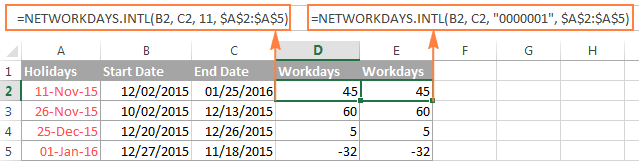## How to highlight workdays in Excel

Using the WORKDAY and WORKDAY.INTL functions, you can not only calculate workdays in your Excel worksheets but also highlight them as your business logic requires. For this, you create a conditional formatting rule with either a WORKDAY or WORKDAY.INTL formula.

For example, in a list of dates in column B, let's highlight only future dates that are within 15 workdays from today's date, excluding two holidays in cells A2:A3. The most obvious formula that comes to mind is as follows:

`=AND(\$B2>TODAY(), \$B2<=WORKDAY(TODAY(), 15, \$A\$2:\$A\$3))`

The first part of the logical test cuts off past dates, i.e. you check if a date is equal to or greater than today: \$B2>TODAY(). And in the second part, you verify whether a date is no more than 15 weekdays in the future, excluding the weekend days and specified holidays: `\$B2<=WORKDAY(TODAY(), 15, \$A\$2:\$A\$3)`

The formula looks correct, but once you create a rule based on it, you will realize that it highlights wrong dates: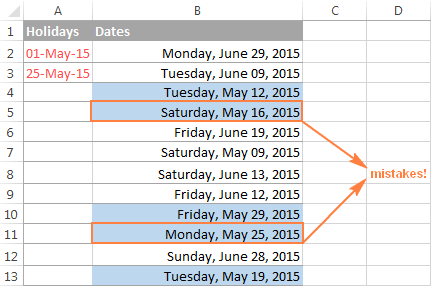Let's try to figure out why that happens. The problem is not with the WORKDAY function, as someone may conclude. The function is right, but... what does it actually do? It returns a date 15 workdays from now, excluding weekend days (Saturday and Sunday) and holidays in cells A2:A3.

Okay, and what does the rule based on this formula do? It highlights ALL the dates that are equal to or greater than today and less than the date returned by the WORKDAY function. You see? All the dates! If you don't want to color the weekends and holidays, then you need to explicitly tell Excel not to. So, we are adding two more conditions to our formula:

As demonstrated in the below screenshot, the improved formula works perfectly:

`=AND(\$B2>TODAY(), \$B2<=WORKDAY(TODAY(), 15, \$A\$2:\$A\$3), COUNTIF(\$A\$2:\$A\$3, \$B2)=0, WEEKDAY(\$B2, 2)<6)`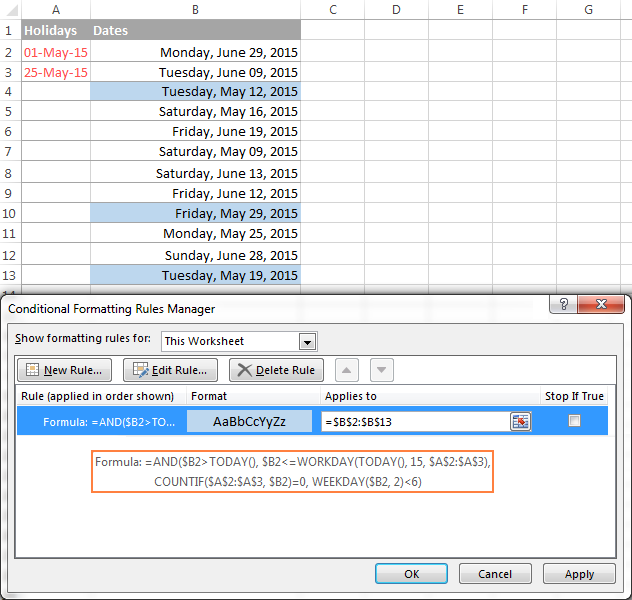As you see, the WORKDAY and WORKDAY.INTL functions make calculating workdays in Excel quick and easy. Of course, your real-life formulas are likely to be more sophisticated, but knowing the basics helps immensely, because you can remember only a small set of essential things and derive the rest. I thank you for reading and hope to see on our blog next week!